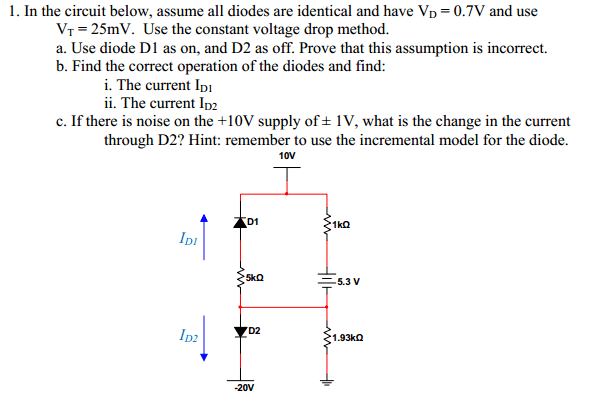# A diode problem

## Homework Statement## The Attempt at a Solution

I know this might seem like a stupid question but are the 10/-20V voltage sources like the 5.3V or are they VDD (which is something different?) I'm also stuck on part a. If D2 is off, that means its not conducting, so zero current, but would there still be a voltage drop across it? Then since its -20V on one side does that mean V(D2)=20V gain to the node on top?

Last edited:

gneill
Mentor

## The Attempt at a Solution

I know this might seem like a stupid question but are the 10/-20V voltage sources like the 5.3V or are they VDD (which is something different?) I'm also stuck on part a. If D2 is off, that means its not conducting, so zero current, but would there still be a voltage drop across it? Then since its -20V on one side does that mean V(D2)=20V gain to the node on top?

Think of the 10/-20V sources as batteries which connect to the indicated terminals and then to ground.

If you are told to assume that a diode is off, simply remove it from the circuit (it supposedly conducts no current so is equivalent to an open circuit. If you are told to assume that a diode is on, for a first pass analysis replace it with a battery equivalent to the junction forward bias (0.7V in this case).

OK, I guess I can treat the 10V as a voltage source that is grounded then. So it won't be part of the loop equation, then I do I(D1)=(5.3+V(D1))/6k where V(D1)=0.7, I(D1)=-1mA. Since there can't be negative current, D1 on/D2 off doesn't work. D1/D2 both off obviously doesn't work either. When I simulated the circuit for D1 off/D2 on I got I(D2)=34mA. is this the answer?

Last edited:
gneill
Mentor
OK, I guess I can treat the 10V as a voltage source that is grounded then. So it won't be part of the loop equation, then I do I(D1)=(5.3+V(D1))/6k where V(D1)=0.7, I(D1)=-1mA. Since there can't be negative current, D1 on/D2 off doesn't work. D1/D2 both off obviously doesn't work either. When I simulated the circuit for D1 off/D2 on I got I(D2)=34mA. is this the answer?

You can't ignore the 10V supply because it has a path through your loop to ground via the 1.93 kΩ resistor. In effect you have a second loop (stick a battery in there for the 10V supply and wire its negative lead to the ground symbol at the bottom to "see" the loop).

You might find that nodal analysis will treat you well here. Only one node to worry about!

Your simulation yields the correct current for ID2 (it's what I've just calculated).